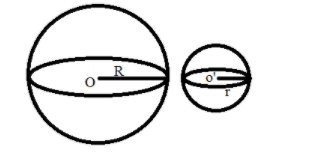QuestionAnswers

# If a spherical steel ball is melted to make 8 new identical balls then the radius of the new ball is how many times the radius of the original ball?Verified
129k+ views
Hint: In this particular question use the concept that the volume of the spherical steel ball is equal to the volume of the 8 new identical balls and the volume of the sphere is $\dfrac{4}{3}\pi {r^3}$, so use these concepts to reach the solution of the question.Consider a spherical steel ball with center O having radius R is shown in the above figure.
Now this spherical steel ball is melted into 8 new identical balls, one of the new steel balls is shown in the above figure having center O’, and radius r.
Now as we know that the volume of the sphere is $\dfrac{4}{3}\pi {R^3}$ cubic units, where R is the radius of the spherical ball.
As we know that when we melted the spherical steel ball to make 8 new identical balls the volume remains constant.
So the volume of the big spherical ball = volume of the 8 new spherical balls.
$\Rightarrow \dfrac{4}{3}\pi {R^3} = 8\left( {\dfrac{4}{3}\pi {r^3}} \right)$
Where R is the radius of the big spherical steel ball and r is the radius of the new spherical steel balls.
Now simplify it we have,
$\Rightarrow {R^3} = 8\left( {{r^3}} \right)$
$\Rightarrow {r^3} = \dfrac{{{R^3}}}{8}$
$\Rightarrow {r^3} = \dfrac{{{R^3}}}{{{2^3}}}$
Now take cube root on both sides we have,
$\Rightarrow r = \sqrt{{\dfrac{{{R^3}}}{{{2^3}}}}} = \dfrac{R}{2}$
So the radius of the new balls is half of the radius of the original spherical steel balls.

Note: Whenever we face such types of questions the key concept we have to remember is that always recall the when we melted something to make a new thing volume always remain constant and always recall the formula of the volume of the sphere which is stated above so simply equate the volume of the original spherical steel ball with the volume of the 8 new identical balls as above and simplify we will get the required radius of the new ball in terms of original ball.